# 12.2 The hyperbola  (Page 9/13)

 Page 9 / 13

$\frac{{\left(y-6\right)}^{2}}{36}-\frac{{\left(x+1\right)}^{2}}{16}=1$

$\frac{{\left(x-2\right)}^{2}}{49}-\frac{{\left(y+7\right)}^{2}}{49}=1$

$\frac{{\left(x-2\right)}^{2}}{{7}^{2}}-\frac{{\left(y+7\right)}^{2}}{{7}^{2}}=1;\text{\hspace{0.17em}}$ vertices: $\text{\hspace{0.17em}}\left(9,-7\right),\left(-5,-7\right);\text{\hspace{0.17em}}$ foci: $\text{\hspace{0.17em}}\left(2+7\sqrt{2},-7\right),\left(2-7\sqrt{2},-7\right);\text{\hspace{0.17em}}$ asymptotes: $\text{\hspace{0.17em}}y=x-9,y=-x-5$

$4{x}^{2}-8x-9{y}^{2}-72y+112=0$

$-9{x}^{2}-54x+9{y}^{2}-54y+81=0$

$\frac{{\left(x+3\right)}^{2}}{{3}^{2}}-\frac{{\left(y-3\right)}^{2}}{{3}^{2}}=1;\text{\hspace{0.17em}}$ vertices: $\text{\hspace{0.17em}}\left(0,3\right),\left(-6,3\right);\text{\hspace{0.17em}}$ foci: $\text{\hspace{0.17em}}\left(-3+3\sqrt{2},1\right),\left(-3-3\sqrt{2},1\right);\text{\hspace{0.17em}}$ asymptotes: $\text{\hspace{0.17em}}y=x+6,y=-x$

$4{x}^{2}-24x-36{y}^{2}-360y+864=0$

$-4{x}^{2}+24x+16{y}^{2}-128y+156=0$

$\frac{{\left(y-4\right)}^{2}}{{2}^{2}}-\frac{{\left(x-3\right)}^{2}}{{4}^{2}}=1;\text{\hspace{0.17em}}$ vertices: $\text{\hspace{0.17em}}\left(3,6\right),\left(3,2\right);\text{\hspace{0.17em}}$ foci: $\text{\hspace{0.17em}}\left(3,4+2\sqrt{5}\right),\left(3,4-2\sqrt{5}\right);\text{\hspace{0.17em}}$ asymptotes: $\text{\hspace{0.17em}}y=\frac{1}{2}\left(x-3\right)+4,y=-\frac{1}{2}\left(x-3\right)+4$

$-4{x}^{2}+40x+25{y}^{2}-100y+100=0$

${x}^{2}+2x-100{y}^{2}-1000y+2401=0$

$\frac{{\left(y+5\right)}^{2}}{{7}^{2}}-\frac{{\left(x+1\right)}^{2}}{{70}^{2}}=1;\text{\hspace{0.17em}}$ vertices: $\text{\hspace{0.17em}}\left(-1,2\right),\left(-1,-12\right);\text{\hspace{0.17em}}$ foci: $\text{\hspace{0.17em}}\left(-1,-5+7\sqrt{101}\right),\left(-1,-5-7\sqrt{101}\right);\text{\hspace{0.17em}}$ asymptotes: $\text{\hspace{0.17em}}y=\frac{1}{10}\left(x+1\right)-5,y=-\frac{1}{10}\left(x+1\right)-5$

$-9{x}^{2}+72x+16{y}^{2}+16y+4=0$

$4{x}^{2}+24x-25{y}^{2}+200y-464=0$

$\frac{{\left(x+3\right)}^{2}}{{5}^{2}}-\frac{{\left(y-4\right)}^{2}}{{2}^{2}}=1;\text{\hspace{0.17em}}$ vertices: $\text{\hspace{0.17em}}\left(2,4\right),\left(-8,4\right);\text{\hspace{0.17em}}$ foci: $\text{\hspace{0.17em}}\left(-3+\sqrt{29},4\right),\left(-3-\sqrt{29},4\right);\text{\hspace{0.17em}}$ asymptotes: $\text{\hspace{0.17em}}y=\frac{2}{5}\left(x+3\right)+4,y=-\frac{2}{5}\left(x+3\right)+4$

For the following exercises, find the equations of the asymptotes for each hyperbola.

$\frac{{y}^{2}}{{3}^{2}}-\frac{{x}^{2}}{{3}^{2}}=1$

$\frac{{\left(x-3\right)}^{2}}{{5}^{2}}-\frac{{\left(y+4\right)}^{2}}{{2}^{2}}=1$

$y=\frac{2}{5}\left(x-3\right)-4,y=-\frac{2}{5}\left(x-3\right)-4$

$\frac{{\left(y-3\right)}^{2}}{{3}^{2}}-\frac{{\left(x+5\right)}^{2}}{{6}^{2}}=1$

$9{x}^{2}-18x-16{y}^{2}+32y-151=0$

$y=\frac{3}{4}\left(x-1\right)+1,y=-\frac{3}{4}\left(x-1\right)+1$

$16{y}^{2}+96y-4{x}^{2}+16x+112=0$

## Graphical

For the following exercises, sketch a graph of the hyperbola, labeling vertices and foci.

$\frac{{x}^{2}}{49}-\frac{{y}^{2}}{16}=1$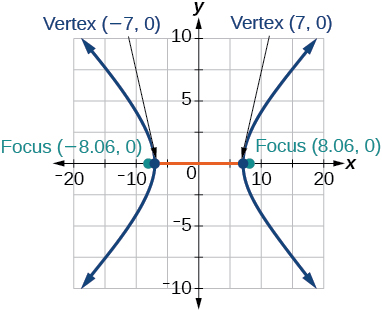$\frac{{x}^{2}}{64}-\frac{{y}^{2}}{4}=1$

$\frac{{y}^{2}}{9}-\frac{{x}^{2}}{25}=1$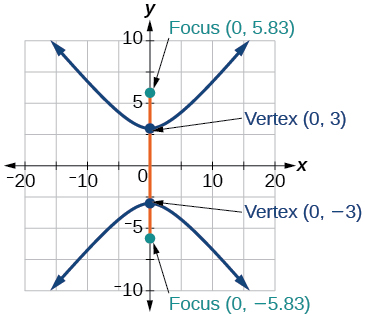$81{x}^{2}-9{y}^{2}=1$

$\frac{{\left(y+5\right)}^{2}}{9}-\frac{{\left(x-4\right)}^{2}}{25}=1$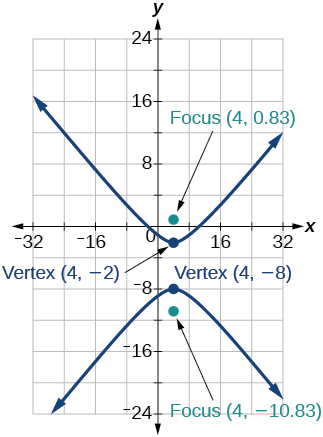$\frac{{\left(x-2\right)}^{2}}{8}-\frac{{\left(y+3\right)}^{2}}{27}=1$

$\frac{{\left(y-3\right)}^{2}}{9}-\frac{{\left(x-3\right)}^{2}}{9}=1$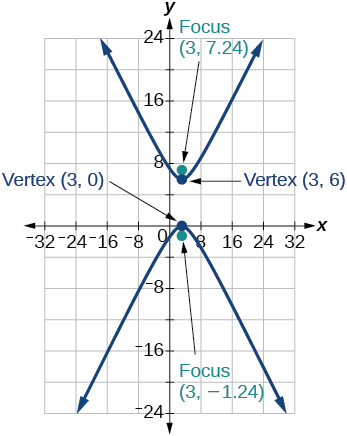$-4{x}^{2}-8x+16{y}^{2}-32y-52=0$

${x}^{2}-8x-25{y}^{2}-100y-109=0$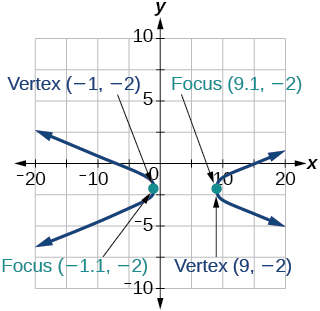$-{x}^{2}+8x+4{y}^{2}-40y+88=0$

$64{x}^{2}+128x-9{y}^{2}-72y-656=0$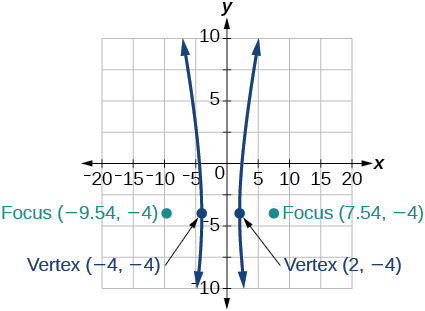$16{x}^{2}+64x-4{y}^{2}-8y-4=0$

$-100{x}^{2}+1000x+{y}^{2}-10y-2575=0$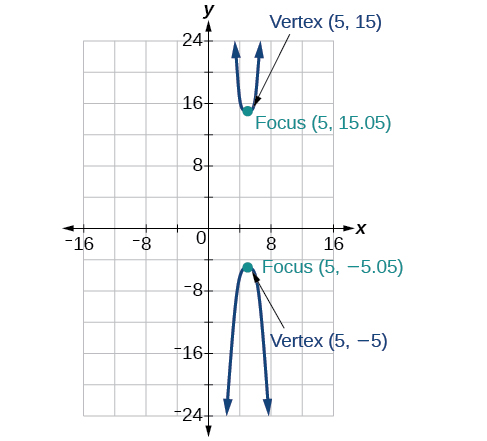$4{x}^{2}+16x-4{y}^{2}+16y+16=0$

For the following exercises, given information about the graph of the hyperbola, find its equation.

Vertices at $\text{\hspace{0.17em}}\left(3,0\right)\text{\hspace{0.17em}}$ and $\text{\hspace{0.17em}}\left(-3,0\right)\text{\hspace{0.17em}}$ and one focus at $\text{\hspace{0.17em}}\left(5,0\right).$

$\frac{{x}^{2}}{9}-\frac{{y}^{2}}{16}=1$

Vertices at $\text{\hspace{0.17em}}\left(0,6\right)\text{\hspace{0.17em}}$ and $\text{\hspace{0.17em}}\left(0,-6\right)\text{\hspace{0.17em}}$ and one focus at $\text{\hspace{0.17em}}\left(0,-8\right).$

Vertices at $\text{\hspace{0.17em}}\left(1,1\right)\text{\hspace{0.17em}}$ and $\text{\hspace{0.17em}}\left(11,1\right)\text{\hspace{0.17em}}$ and one focus at $\text{\hspace{0.17em}}\left(12,1\right).$

$\frac{{\left(x-6\right)}^{2}}{25}-\frac{{\left(y-1\right)}^{2}}{11}=1$

Center: $\text{\hspace{0.17em}}\left(0,0\right);$ vertex: $\text{\hspace{0.17em}}\left(0,-13\right);$ one focus: $\text{\hspace{0.17em}}\left(0,\sqrt{313}\right).$

Center: $\text{\hspace{0.17em}}\left(4,2\right);$ vertex: $\text{\hspace{0.17em}}\left(9,2\right);$ one focus: $\text{\hspace{0.17em}}\left(4+\sqrt{26},2\right).$

$\frac{{\left(x-4\right)}^{2}}{25}-\frac{{\left(y-2\right)}^{2}}{1}=1$

Center: $\text{\hspace{0.17em}}\left(3,5\right);\text{\hspace{0.17em}}$ vertex: $\text{\hspace{0.17em}}\left(3,11\right);\text{\hspace{0.17em}}$ one focus: $\text{\hspace{0.17em}}\left(3,5+2\sqrt{10}\right).$

For the following exercises, given the graph of the hyperbola, find its equation.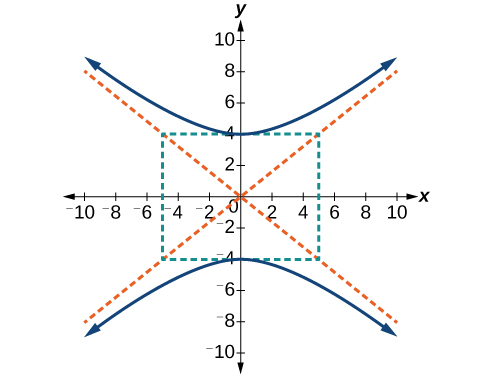$\frac{{y}^{2}}{16}-\frac{{x}^{2}}{25}=1$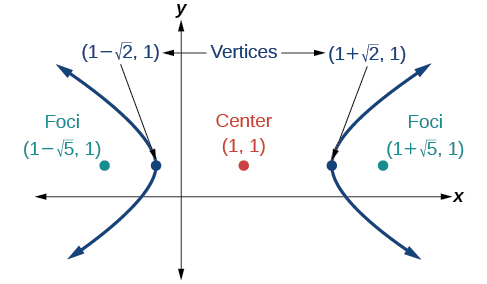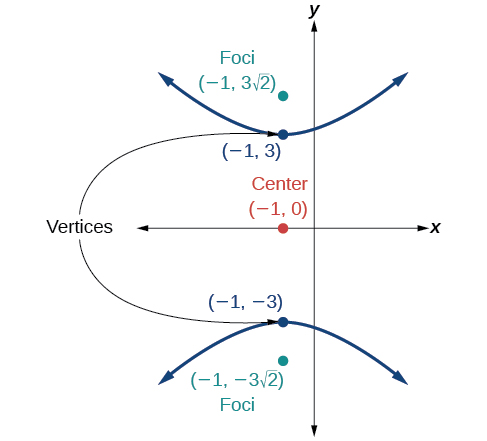$\frac{{y}^{2}}{9}-\frac{{\left(x+1\right)}^{2}}{9}=1$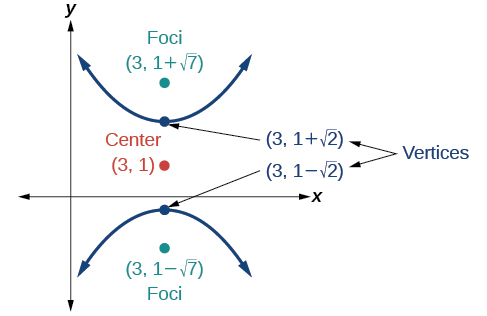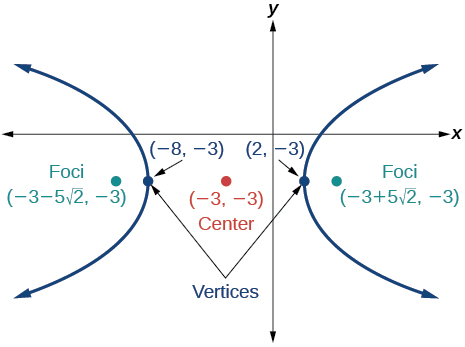$\frac{{\left(x+3\right)}^{2}}{25}-\frac{{\left(y+3\right)}^{2}}{25}=1$

## Extensions

For the following exercises, express the equation for the hyperbola as two functions, with $\text{\hspace{0.17em}}y\text{\hspace{0.17em}}$ as a function of $\text{\hspace{0.17em}}x.\text{\hspace{0.17em}}$ Express as simply as possible. Use a graphing calculator to sketch the graph of the two functions on the same axes.

$\frac{{x}^{2}}{4}-\frac{{y}^{2}}{9}=1$

$\frac{{y}^{2}}{9}-\frac{{x}^{2}}{1}=1$

$y\left(x\right)=3\sqrt{{x}^{2}+1},y\left(x\right)=-3\sqrt{{x}^{2}+1}$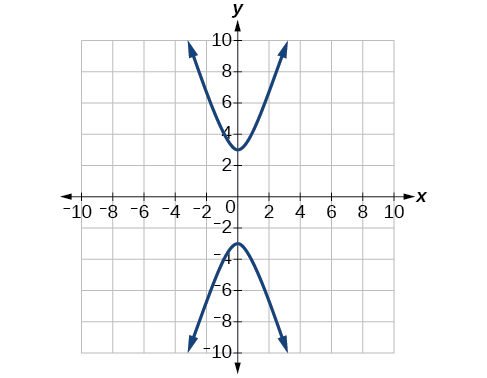$\frac{{\left(x-2\right)}^{2}}{16}-\frac{{\left(y+3\right)}^{2}}{25}=1$

$-4{x}^{2}-16x+{y}^{2}-2y-19=0$

$y\left(x\right)=1+2\sqrt{{x}^{2}+4x+5},y\left(x\right)=1-2\sqrt{{x}^{2}+4x+5}$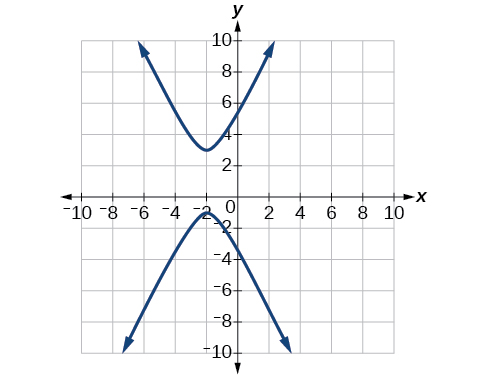$4{x}^{2}-24x-{y}^{2}-4y+16=0$

## Real-world applications

For the following exercises, a hedge is to be constructed in the shape of a hyperbola near a fountain at the center of the yard. Find the equation of the hyperbola and sketch the graph.

The hedge will follow the asymptotes and its closest distance to the center fountain is 5 yards.

$\frac{{x}^{2}}{25}-\frac{{y}^{2}}{25}=1$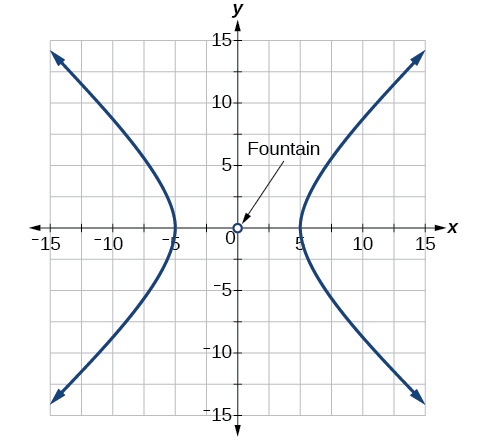The hedge will follow the asymptotes and its closest distance to the center fountain is 6 yards.

The hedge will follow the asymptotes $\text{\hspace{0.17em}}y=\frac{1}{2}x\text{\hspace{0.17em}}$ and $\text{\hspace{0.17em}}y=-\frac{1}{2}x,$ and its closest distance to the center fountain is 10 yards.

$\frac{{x}^{2}}{100}-\frac{{y}^{2}}{25}=1$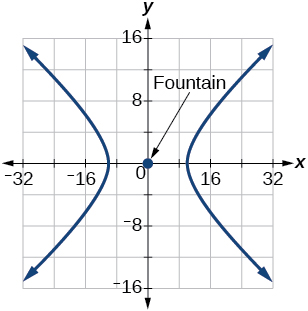The hedge will follow the asymptotes $\text{\hspace{0.17em}}y=\frac{2}{3}x\text{\hspace{0.17em}}$ and $\text{\hspace{0.17em}}y=-\frac{2}{3}x,$ and its closest distance to the center fountain is 12 yards.

The hedge will follow the asymptotes and its closest distance to the center fountain is 20 yards.

$\frac{{x}^{2}}{400}-\frac{{y}^{2}}{225}=1$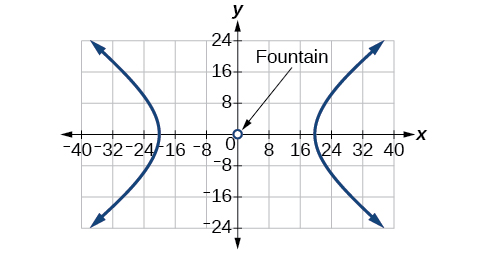For the following exercises, assume an object enters our solar system and we want to graph its path on a coordinate system with the sun at the origin and the x-axis as the axis of symmetry for the object's path. Give the equation of the flight path of each object using the given information.

The object enters along a path approximated by the line $\text{\hspace{0.17em}}y=x-2\text{\hspace{0.17em}}$ and passes within 1 au (astronomical unit) of the sun at its closest approach, so that the sun is one focus of the hyperbola. It then departs the solar system along a path approximated by the line $\text{\hspace{0.17em}}y=-x+2.\text{\hspace{0.17em}}$

The object enters along a path approximated by the line $\text{\hspace{0.17em}}y=2x-2\text{\hspace{0.17em}}$ and passes within 0.5 au of the sun at its closest approach, so the sun is one focus of the hyperbola. It then departs the solar system along a path approximated by the line $\text{\hspace{0.17em}}y=-2x+2.\text{\hspace{0.17em}}$

$\frac{{\left(x-1\right)}^{2}}{0.25}-\frac{{y}^{2}}{0.75}=1$

The object enters along a path approximated by the line $\text{\hspace{0.17em}}y=0.5x+2\text{\hspace{0.17em}}$ and passes within 1 au of the sun at its closest approach, so the sun is one focus of the hyperbola. It then departs the solar system along a path approximated by the line $\text{\hspace{0.17em}}y=-0.5x-2.\text{\hspace{0.17em}}$

The object enters along a path approximated by the line $\text{\hspace{0.17em}}y=\frac{1}{3}x-1\text{\hspace{0.17em}}$ and passes within 1 au of the sun at its closest approach, so the sun is one focus of the hyperbola. It then departs the solar system along a path approximated by the line

$\frac{{\left(x-3\right)}^{2}}{4}-\frac{{y}^{2}}{5}=1$

The object It enters along a path approximated by the line $\text{\hspace{0.17em}}y=3x-9\text{\hspace{0.17em}}$ and passes within 1 au of the sun at its closest approach, so the sun is one focus of the hyperbola. It then departs the solar system along a path approximated by the line $\text{\hspace{0.17em}}y=-3x+9.\text{\hspace{0.17em}}$

(x2-2x+8)-4(x2-3x+5)
sorry
Miranda
x²-2x+9-4x²+12x-20 -3x²+10x+11
Miranda
x²-2x+9-4x²+12x-20 -3x²+10x+11
Miranda
(X2-2X+8)-4(X2-3X+5)=0 ?
master
The anwser is imaginary number if you want to know The anwser of the expression you must arrange The expression and use quadratic formula To find the answer
master
The anwser is imaginary number if you want to know The anwser of the expression you must arrange The expression and use quadratic formula To find the answer
master
Y
master
master
Soo sorry (5±Root11* i)/3
master
Mukhtar
explain and give four example of hyperbolic function
What is the correct rational algebraic expression of the given "a fraction whose denominator is 10 more than the numerator y?
y/y+10
Mr
Find nth derivative of eax sin (bx + c).
Find area common to the parabola y2 = 4ax and x2 = 4ay.
Anurag
A rectangular garden is 25ft wide. if its area is 1125ft, what is the length of the garden
to find the length I divide the area by the wide wich means 1125ft/25ft=45
Miranda
thanks
Jhovie
What do you call a relation where each element in the domain is related to only one value in the range by some rules?
A banana.
Yaona
given 4cot thither +3=0and 0°<thither <180° use a sketch to determine the value of the following a)cos thither
what are you up to?
nothing up todat yet
Miranda
hi
jai
hello
jai
Miranda Drice
jai
aap konsi country se ho
jai
which language is that
Miranda
I am living in india
jai
good
Miranda
what is the formula for calculating algebraic
I think the formula for calculating algebraic is the statement of the equality of two expression stimulate by a set of addition, multiplication, soustraction, division, raising to a power and extraction of Root. U believe by having those in the equation you will be in measure to calculate it
Miranda
state and prove Cayley hamilton therom
hello
Propessor
hi
Miranda
the Cayley hamilton Theorem state if A is a square matrix and if f(x) is its characterics polynomial then f(x)=0 in another ways evey square matrix is a root of its chatacteristics polynomial.
Miranda
hi
jai
hi Miranda
jai
thanks
Propessor
welcome
jai
What is algebra
algebra is a branch of the mathematics to calculate expressions follow.
Miranda
Miranda Drice would you mind teaching me mathematics? I think you are really good at math. I'm not good at it. In fact I hate it. 😅😅😅
Jeffrey
lolll who told you I'm good at it
Miranda
something seems to wispher me to my ear that u are good at it. lol
Jeffrey
lolllll if you say so
Miranda
but seriously, Im really bad at math. And I hate it. But you see, I downloaded this app two months ago hoping to master it.
Jeffrey
which grade are you in though
Miranda
oh woww I understand
Miranda
Jeffrey
Jeffrey
Miranda
how come you finished in college and you don't like math though
Miranda
gotta practice, holmie
Steve
if you never use it you won't be able to appreciate it
Steve
I don't know why. But Im trying to like it.
Jeffrey
yes steve. you're right
Jeffrey
so you better
Miranda
what is the solution of the given equation?
which equation
Miranda
I dont know. lol
Jeffrey
Miranda
Jeffrey
answer and questions in exercise 11.2 sums
how do u calculate inequality of irrational number?
Alaba
give me an example
Chris
and I will walk you through it
Chris
cos (-z)= cos z .
cos(- z)=cos z
Mustafa
what is a algebra
(x+x)3=?
6x
Obed
what is the identity of 1-cos²5x equal to?
__john __05
Kishu
Hi
Abdel
hi
Ye
hi
Nokwanda
C'est comment
Abdel
Hi
Amanda
hello
SORIE
Hiiii
Chinni
hello
Ranjay
hi
ANSHU
hiiii
Chinni
h r u friends
Chinni
yes
Hassan
so is their any Genius in mathematics here let chat guys and get to know each other's
SORIE
I speak French
Abdel
okay no problem since we gather here and get to know each other
SORIE
hi im stupid at math and just wanna join here
Yaona
lol nahhh none of us here are stupid it's just that we have Fast, Medium, and slow learner bro but we all going to work things out together
SORIE
it's 12ByByBy Brooke DelaneyBy JavaChamp TeamBy OpenStaxBy Anonymous UserBy Mackenzie WilcoxBy Richley CrapoBy JavaChamp TeamByBy Madison ChristianBy OpenStax